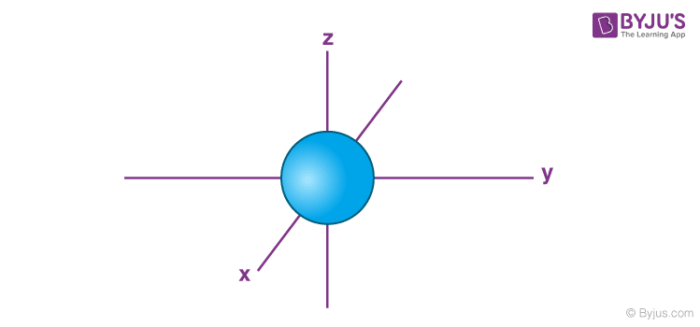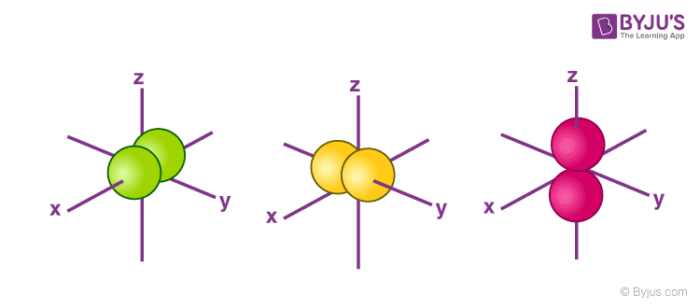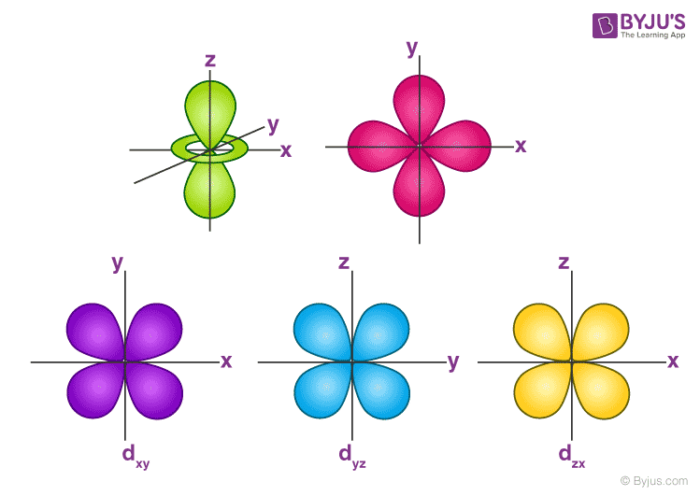# Orbitals Chemistry

## What are Atomic Orbitals?

Atomic orbitals are mathematical functions that describe the wave nature of electrons (or electron pairs) in an atom.

They offer a way to calculate the probability of finding an electron in a specified region around the nucleus of the atom.

## Orbitals Chemistry

There are four different kinds of orbitals, denoted s, p, d and f each with a different shape. Of the four, s and p orbitals are considered because these orbitals are the most common in organic and biological chemistry. An s-orbital is spherical with the nucleus at its centre, a p-orbitals is dumbbell-shaped and four of the five d orbitals are cloverleaf shaped. The fifth d orbital is shaped like an elongated dumbbell with a doughnut around its middle. The orbitals in an atom are organized into different layers or electron shells.

## Recommended Videos

#### Shapes of Orbitals#### What is an Orbital?According to the quantum atomic model, an atom can have many possible numbers of orbitals. These orbitals can be categorized on the basis of their size, shape or orientation. A smaller sized orbital means there is a greater chance of getting an electron near the nucleus. The orbital wave function or ϕ is a mathematical function used for representing the coordinates of an electron. The square of the orbital wave function represents the probability of finding an electron.

This wave function also helps us in drawing boundary surface diagrams. Boundary surface diagrams of the constant probability density for different orbitals help us understand the shape of orbitals.

Let us represent the shapes of orbitals with the help of boundary surface diagrams:

## The Shape of s Orbitals

• The boundary surface diagram for the s orbital looks like a sphere having the nucleus as its centre which in two dimensions can be seen as a circle.
• Hence, we can say that s-orbitals are spherically symmetric having the probability of finding the electron at a given distance equal in all the directions.
• The size of the s orbital is also found to increase with the increase in the value of the principal quantum number (n), thus, 4s > 3s> 2s > 1s.## The Shape of p Orbitals

• Each p orbital consists of two sections better known as lobes which lie on either side of the plane passing through the nucleus.
• The three p orbitals differ in the way the lobes are oriented whereas they are identical in terms of size, shape, and energy.
• As the lobes lie along one of the x, y or z-axis, these three orbitals are given the designations 2px, 2py, and 2pz. Thus, we can say that there are three p orbitals whose axes are mutually perpendicular.
• Similar to s orbitals the size, and energy of p orbitals increase with an increase in the principal quantum number (4p > 3p > 2p).## The Shape of d Orbitals

• The magnetic orbital quantum number for d orbitals is given as (-2,-1,0, 1,2). Hence, we can say that there are five d-orbitals.
• These orbitals are designated as dxy, dyz, dxz, dx2–y 2 and dz2.
• Out of these five d orbitals, the shapes of the first four d-orbitals are similar to each other, which is different from the dz2 orbital whereas the energy of all five d orbitals is the same.## Solved Example

Assuming 2s-2p mixing is NOT operative, the paramagnetic species among the following is :
(A) Be2
(B) B2
(C) C2
(D) N2

Solution:

C2 = valence electrons =8
(σ2s)2(σ∗2s)2(σ2pz)2(π2px)1(π2py)1
Because if the question says 2s-2p orbital intermixing is not operative it indicates you to fill the molecular orbitals according to the energy order of the system having electrons >14
The electrons are unpaired in a bonding molecular orbital. Rest in all other molecules the electrons are paired all others are diamagnetic.

## Frequently Asked Questions – FAQs

Q1

### How many orbitals are there in chemistry?

The four different orbital forms (s, p, d, and f) have different sizes and one orbital will accommodate up to two electrons at most. The orbitals p, d, and f have separate sub-levels and will thus accommodate more electrons. As shown, each element’s electron configuration is unique to its position on the periodic table.

Q2

### How do orbitals work?

An atomic orbital is a mathematical term in atomic theory and quantum mechanics that describes the wave-like behaviour of either one electron or a pair of electrons in an atom. Every such orbital will occupy a maximum of two electrons, each having its own quantity of spin.

Q3

### How many orbitals are there?

The s sublevel has only one orbital, so max. 2 electrons can be present. The p sublevel has 3 orbitals, so max. 6 electrons can be present. The d sublevel has 5 orbitals, so max. 10 electrons can be present. And the 4 sub-levels have seven orbitals, and they can hold max of 14 electrons.

Q4

### Why is the s orbital spherical?

All s orbitals are shaped spherically and have spherical symmetry. That means the function of the wave will depend only on the distance from the nucleus and not on the direction. For any particle, as the central quantum number of the orbital decreases, the size of the orbital decreases, but the geometry stays spherical.

Q5

### What is sigma and pi bond?

Sigma and pi bonds are formed by atomic orbital overlap. Sigma bonds are formed by overlapping end-to-end and Pi bonds occur where one atomic orbital lobe overlaps another. As seen along the axis of the bond, both derived their names from the Greek letters and the bond.

Q6

### What does P orbital stand for?

The s, p, d, and f, respectively stand for sharp, primary, diffuse and fundamental. The letters and words refer to the visual impression left by the spectral lines’ fine structure that occurs because of the first relativistic corrections, particularly the spin-orbital interaction.

Q7

### Which orbitals have the highest energy?

The orbital 1s hold the highest energy. You will appreciate it by talking of different things: But first let’s be super clear: an electron’s energy is the energy it will take to pull it out of the electrical bubble of the atom.

Q8

### What is the difference between a shell and an orbital?

A shell in an atom is a set of subshells of the same quantum number theory, n. Orbitals contain two electrons each, and electrons are part of the same orbital in an orbital of the same definition of size, angular momentum size, and magnetic quantum number.

Take up a quiz on Orbitals Chemistry